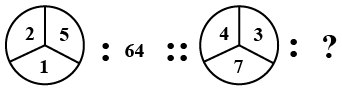### SSC JE Mechanical Engineering 2012 Question 19

Instructions

Select the related word/letter /number/figure from the given alternatives.

Question 19

#Solution

The logic here is

$$\left(2+5+1\right)^2=8^2=64$$

The right number is the square of sum of the numbers in the figure.

Similarly,

The required number = $$\left(4+3+7\right)^2=14^2=196$$

Hence, the correct answer is Option B